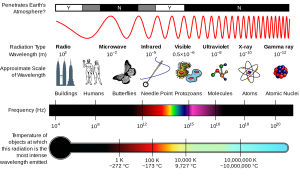# Electromagnetic radiation - Wave nature

## What is Electromagnetic Radiation?

Whenever a charge is placed in an electric or a magnetic field, it experiences a certain force acting on it or if multiple charges are placed, they experience an interaction due to another.

In the year 1870, James Maxwell became the first scientist to explain the interaction between the charges in the presence of electric and magnetic fields. He proposed that when electrically charged particles perform an accelerating motion, alternating electrical and magnetic fields are produced and transmitted. These fields traverse in the form of waves known as electromagnetic radiation. A light wave is an example of electromagnetic radiation.## Properties of Electromagnetic Radiation:

1. The oscillating charged particles produce oscillating electric and magnetic fields which are perpendicular to each other and both are perpendicular to the direction of propagation of the wave.
2. Electromagnetic waves do not require a medium i.e., they can travel in a vacuum too.
3. There are many kinds of electromagnetic radiation, differing from one another in terms of wavelength or frequency. This electromagnetic radiation as a whole constitutes the electromagnetic spectrum. For example radio frequency region, microwave region, infrared region, ultraviolet region, visible region etc.
4. Electromagnetic radiation is characterised based on various properties like frequency, wavelength, time period etc.

## Electromagnetic Radiation Formula

Frequency is defined as the number of waves that pass through a given point in one second. Mathematically it is equal to the reciprocal of the time period of electromagnetic radiation. A general equation relating the speed of light, frequency, and wavelength of electromagnetic radiation is given below:

c = ν 𝝀

Where,

• c= speed of light,
• ν= frequency of the electromagnetic wave and
• 𝝀 = wavelength of the electromagnetic wave.

Apart from frequency and wavelength, some other parameters are also used to categorise electromagnetic radiation. One of these parameters is the wave number. Wave number is defined as the number of wavelengths per unit length. Mathematically, it is equal to the reciprocal of the wavelength. It is expressed in the SI unit as m-1.

## Frequently Asked Questions – FAQs

### What is the wave nature of electromagnetic radiation?

Electromagnetic (EM) radiation is a source of energy which is propagated in the form of electromagnetic waves through free space or through a material medium. The dual existence of electromagnetic radiation: it shows wave and particulate (photon) properties.

### What is the nature of waves?

A longitudinal wave is a disturbance that moves like air or water through a medium. Fluids such as these may be assumed to consist of a huge number of particles” composed of a massive number of molecules each.

### Is electromagnetic radiation harmful to humans?

There is no question that exposure to extremely high levels of electromagnetic fields in the short term can be hazardous to health. Despite thorough research, there is no reason to date to suggest that exposure to electromagnetic fields at low levels is adverse to human health.

### What are electromagnetic waves and their properties?

EM waves ride in a vacuum at a fixed frequency of 3.00 x 108 ms-1. Neither the electric field nor the gravitational field deflects them. They are capable of exhibiting interference or diffraction, though. An electric pulse – be it air, a rigid object or a vacuum – can pass through something.

### Is electromagnetic energy a light?

Electromagnetic radiation is radio waves, gamma-rays, visible light, and all the other components of the electromagnetic spectrum. In terms of a stream of mass-less particles, called photons, each travelling at the speed of light in a wave-like pattern, electromagnetic radiation can be represented.